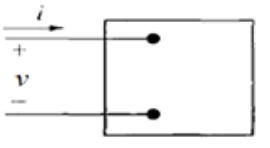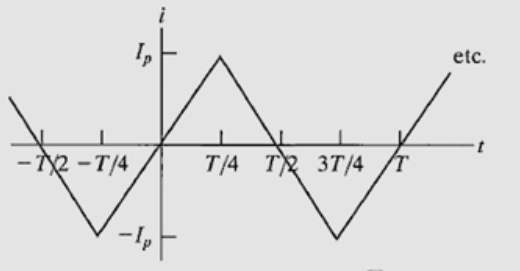Need Help?

Subscribe to Circuit

###### \${selected_topic_name}
• Notes

$\begin{array}{l}{\text { a) Calculate the average power and the reactive power at the terminals of the network shown in if }} \\ {v=100 \cos \left(w+15^{\circ}\right) \mathrm{V}, \mathrm{i}=4 \sin \left(\mathrm{wt}-15^{\circ}\right) \mathrm{A} .} \\ {\text { b) state whether the network inside the box is }} \\ {\text { absorbing or delivering average power. }} \\ {\text { c) State whethr the network inside the box is }} \\ {\text { absorbing or supplying magnetizing vars. }}\end{array}$$V=100 \cos (wt+15)$

$i=4 \sin (w t-15)$

$i=4 \cos [w t-15-90]$

$P=\frac{1}{2} V_{m} I_{m} \cos \left(\theta_v-\theta_{i}\right)$

$P=\frac{1}{2}(100)(4) \cos [15-(-105)]=-100 w$

$Q=\frac{1}{2}V_mI_m \sin (\theta_v-\theta_i)=\frac{1}{2}(100)*(4)*\sin (15+105)$

$Q=173.21 \ VAR\rightarrow (+ve)$

$\begin{array}{l}{\text { The periodic triangular current repeated here, has }} \\ {\text { a peak value of } 180 \mathrm{mA} \text { . }} \\ {\text { Find the average power that this current delivers }} \\ {\text { to a } 5 \mathrm{k} \Omega \text { resistor. }}\end{array}$$P_{VR}=I^{2} R$

$I_{rms}= \frac{I_{\max }}{\sqrt{2}}$        for sine only

$Q=\frac{1}{2}V_mI_m \sin (\theta_v-\theta_i)=\frac{1}{2}(100)*(4)*\sin (15+105)$

$I_{rms}=\frac{180}{\sqrt{3}}=103.9 \ mA$

$p=I_{r m s}^{2} * R$

$P=\left(103.9 * 10^{-3}\right)^2*5*10^3$

$P=54 w$